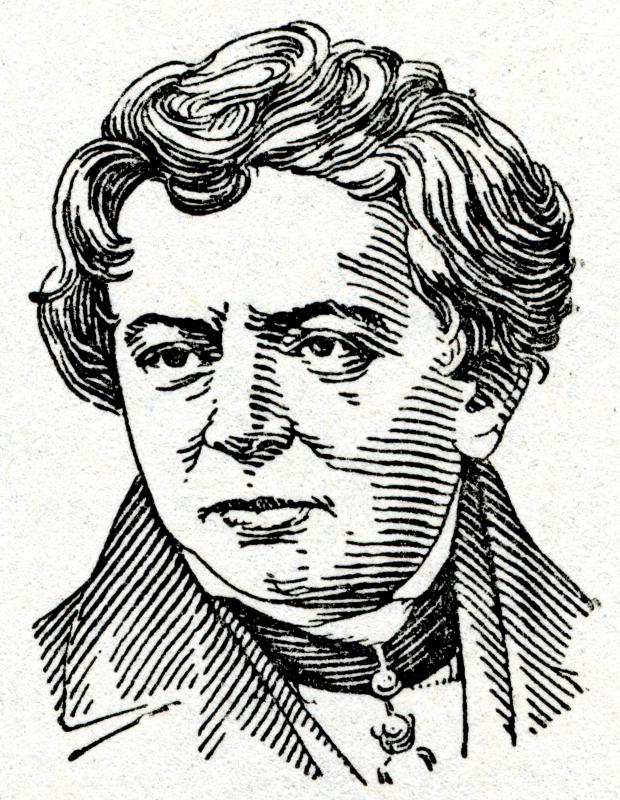# How is Electrical Resistance Measured?

Eric Tallberg

Electrical resistance is a force or material that impedes or resists the free flow of electrical current. Since its discovery by George Ohm in the 19th century, it has been measured in ohms.Electrical resistance was discovered by George Ohm in the 19th century and has since been measured in ohms.

Electricity is basically a flow of electrons. In the case of electrical current, the atoms in the structure of a conductive material either allow or impede the flow of electrons. Poor conductivity means that the atoms are arranged so that they impede, or resist, this flow.Electrical resistance is a force or material that stops or resists the flow of an electrical current.

The actual measurement of electrical resistance (R) is accomplished with the division of voltage (V) by the current flow through an object (I), which is expressed in Siemens: R = V/I. The factor of resistance is expressed in amperes (amps).

The resistance of an object or material — its electrical conductance — is predicated upon both its material construct and its geometrical makeup. Electrical current is either resisted or enabled according to a number of factors, including the quality of insulation, impedance or easement of the conductor, and the density of the object that the current flows through.

There are two basic types of electrical resistance: direct current (DC) resistance and alternating current (AC) resistance. Direct current is the flow of an electrical charge or current in one direction only. Alternating current is a back and forth movement of current.

DC resistance is this unidirectional movement of electrical current encountering the impediment or resistance of its conductor. Using the flow of water through a garden hose, the water would be the current, the water pressure would be the voltage, and the hose would be the conductor. The hose (conductor) enables the unidirectional flow of water (current) within the walls of the hose. The wall provides a measure of friction (resistance), degrading the force of the water flow. In the case of electrical current, insulating material, imperfections, and faults in the electrical conductor create the resistance which the current must overcome.

AC resistance is basically the same as DC resistance except that resistance is encountered in a multidirectional, back and forth, current flow. To use the hose example again, the water in the garden hose could be imagined flowing from and then back to the faucet. Friction (resistance) is therefore multiplied by two.

As mechanical friction creates heat, so does electrical resistance. Just as excessive heat degrades mechanical performance, so it is with electrical performance. Both friction and resistance are, in excess, dangerous and destructive.

## You might also Likeanon338926

A multimeter is much more convenient, because it is composed of both ohmmeter, voltmeter and ammeter, combined as one tool.

allenJo

A useful tool for measuring electric current is an Ohmmeter. It was one of the first products I purchased when I began building electrical circuit projects many years ago. You can plug terminals into sockets in the ohmmeter and then use the leads to touch the different parts of the circuit board you’re trying to test, and read out the resistance in ohms.

I’ve used it to test individual resistors (you can do this while the resistors are still in the circuit board) as well as to test entire circuits. It’s an indispensable tool for anyone working in electronics.